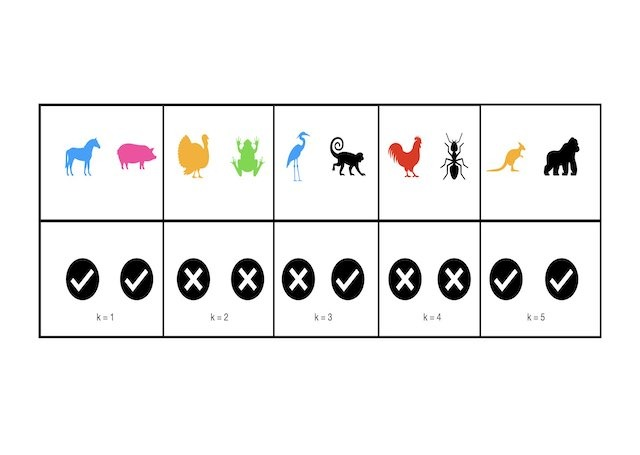## Implement the KNN Algorithm in Python from Scratch

Implement the KNN Algorithm in Python from Scratch In this post, we will cover the K Nearest Neighbours algorithm: how it works …## Implement Gradient Boosting Regression in Python from Scratch

Implement Gradient Boosting Regression in Python from Scratch In this post, we will implement the Gradient Boosting Regression algorithm in Python. This is a …## Understanding the Gradient Boosting Regressor Algorithm

Understanding the Gradient Boosting Regressor Algorithm In this post, we will cover the Gradient Boosting Regressor algorithm: the motivation, foundational assumptions, and derivation …## A Complete Introduction to Cross Validation in Machine Learning

A Complete Introduction to Cross Validation in Machine Learning This post will discuss various Cross Validation techniques. Cross Validation is a testing methodology …## Understanding the Adaboost Regression Algorithm

Understanding the Adaboost Regression Algorithm In this post, we will describe the Adaboost regression algorithm. We will start with the basic assumptions and …## Understanding the Adaboost Classification Algorithm

Understanding the Adaboost Classification Algorithm In this post, we will describe the Adaboost classification algorithm. We will start with the basic assumptions and …